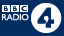# The Imaginary Number

The imaginary number takes mathematics to another dimension. If the square root of +1 is both +1 and -1, then what is the square root of -1?

The imaginary number takes mathematics to another dimension. It was discovered in sixteenth century Italy at a time when being a mathematician was akin to being a modern day rock star, when there was 'nuff respect' to be had from solving a particularly 'wicked' equation. And the wicked equation of the day went like this: 'If the square root of +1 is both +1 and -1, then what is the square root of -1?'

Previously, mathematicians had rolled their eyes skyward and prayed for divine intervention. But where others failed, the creative Italian Rafaello Bombelli triumphed with his invention of the imaginary number. The imaginary number is the square root of -1 and is known as 'i'.

Imaginary numbers are real numbers multiplied by i. If, like many, you find yourself saying 'but what's the point?' then think on this. Imagine a world without electric circuits. No circuits, so no computers. No computers, so you wouldn't be reading this now. And while engineers need the imaginary number to analyse electrical waves, physicists need it to calculate the fundamental forces that govern our Universe via quantum mechanics.

And, most bizarrely of all, time might have an imaginary dimension, which might explain how time started. The Big Bang gave birth to our Universe some 15 billion years ago. It was every mother's dream birth, taking less than a second for all time, matter and energy to be born, with no need for an epidural. But if the Big Bang marks the beginning of time and the beginning of matter, what was there before it? How could time just start?

Einstein's classical general theory of relativity linked together time with the three spatial dimensions we are all familiar with (up - down, left - right, and in - out), creating a four dimensional 'spacetime' in which time can move forwards only. A brilliant theory, but one that comes a cropper when applied to the creation of the Universe.

But invoke quantum theory and a dollop of imaginary time and things begin to make sense ... at least to cosmologists. Imaginary time is measured in imaginary numbers and unlike real time, it can move backwards and forwards like an extra spatial dimension. Apparently, this gives the Big Bang something to start from.

Available now

14 minutes

## Last onThu 14 Mar 2002 15:45

## More episodes

### Next

•